CBSE Class 12 Sample Paper for 2021 Boards

Class 12
Solutions of Sample Papers and Past Year Papers - for Class 12 Boards

## (ii) Let 𝑍=𝑝𝑥 + 𝑞𝑦, where 𝑝,𝑞>𝑜 be the objective function. Find the condition on 𝑝 and 𝑞 so that the maximum value of 𝑍 occurs at B(4,10)𝑎𝑛𝑑 C(6,8). Also mention the number of optimal solutions in this case.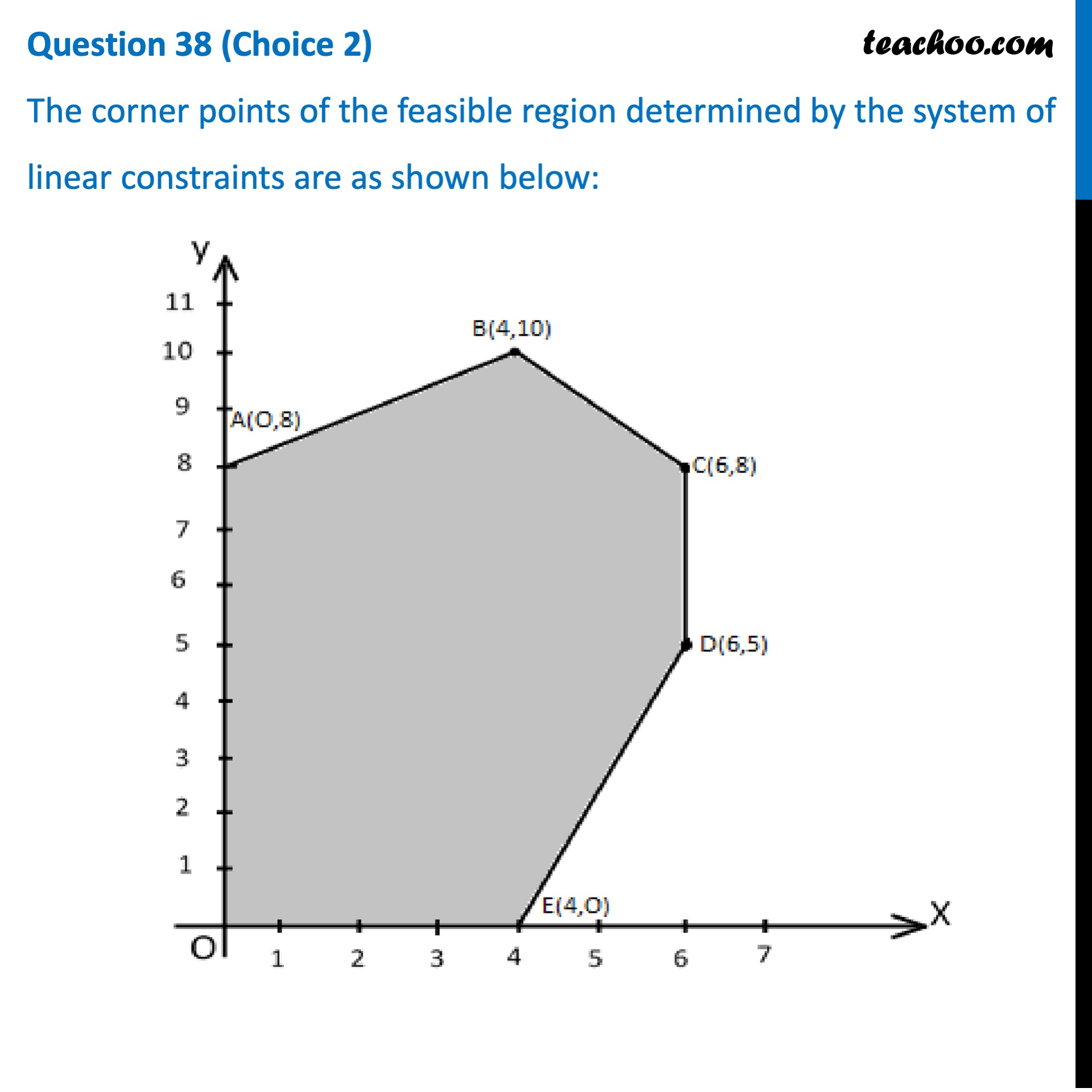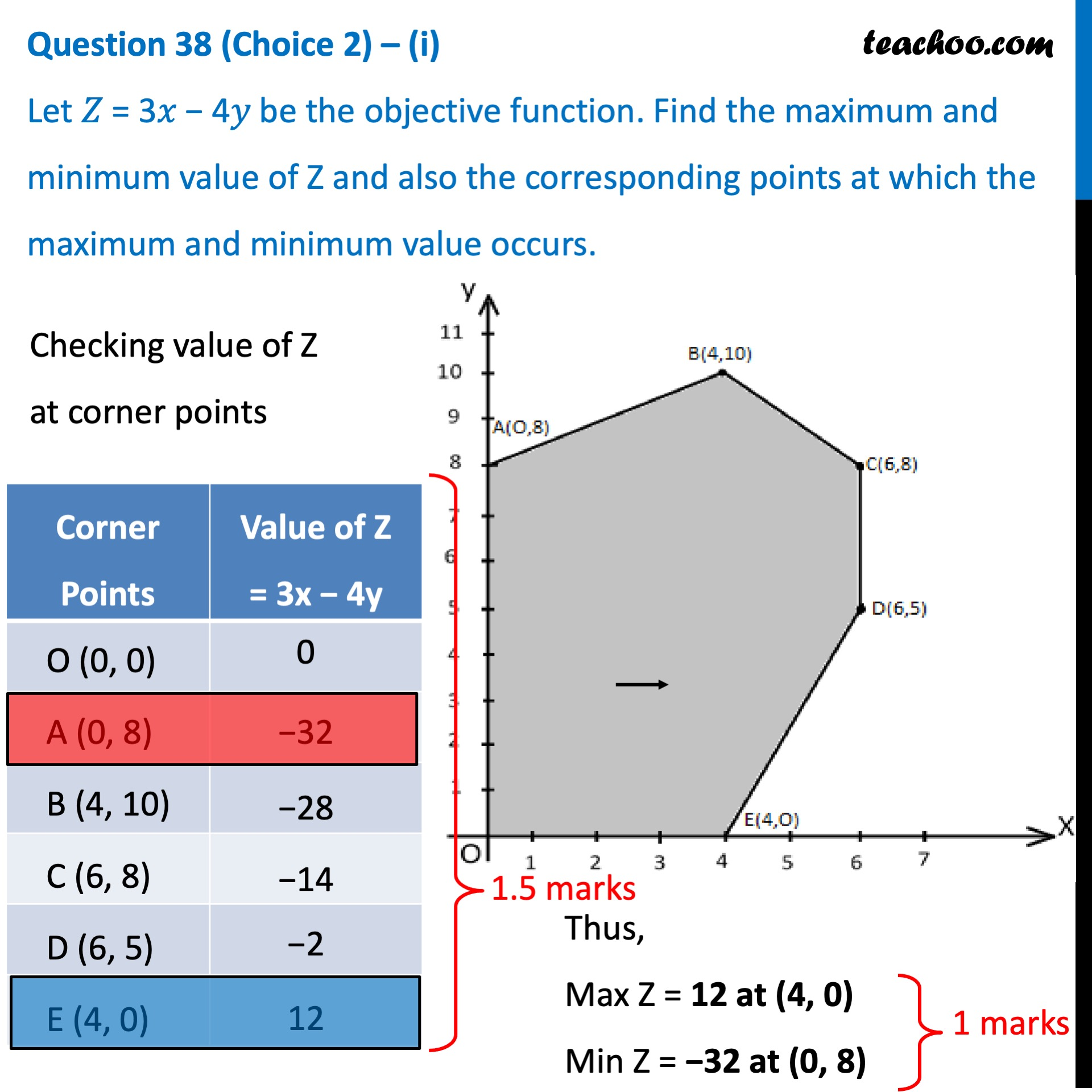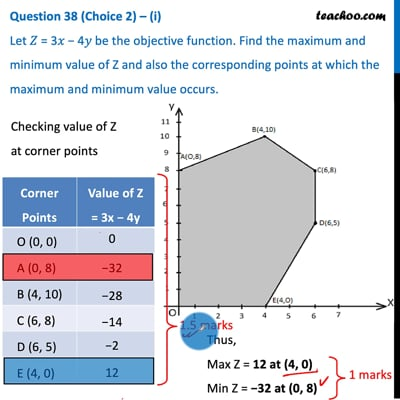This video is only available for Teachoo black users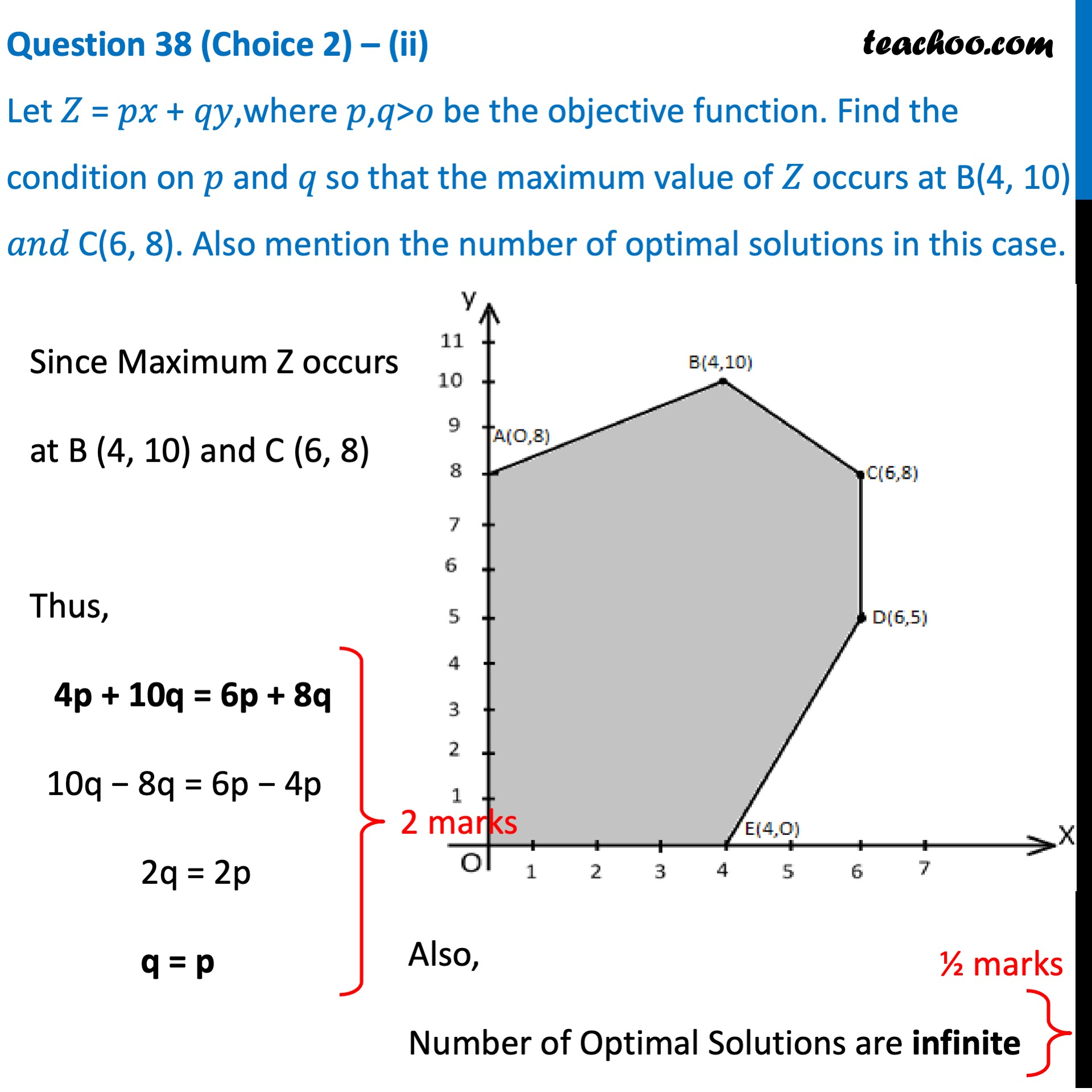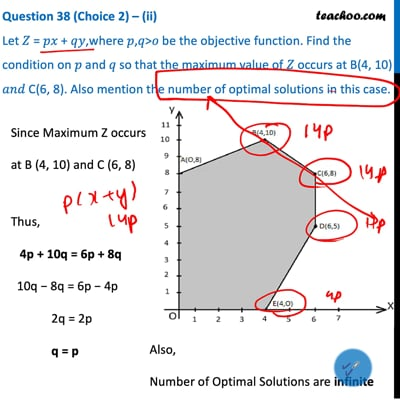This video is only available for Teachoo black users

Introducing your new favourite teacher - Teachoo Black, at only ₹83 per month

### Transcript

Question 38 (Choice 2) The corner points of the feasible region determined by the system of linear constraints are as shown below: Question 38 (Choice 2) – (i) Let 𝑍 = 3𝑥 − 4𝑦 be the objective function. Find the maximum and minimum value of Z and also the corresponding points at which the maximum and minimum value occurs. Checking value of Z at corner points Corner Points Value of Z  = 3x − 4y Thus, Max Z = 12 at (4, 0) Min Z = −32 at (0, 8) Question 38 (Choice 2) – (ii) Let 𝑍 = 𝑝𝑥 + 𝑞𝑦,where 𝑝,𝑞>𝑜 be the objective function. Find the condition on 𝑝 and 𝑞 so that the maximum value of 𝑍 occurs at B(4, 10) 𝑎𝑛𝑑 C(6, 8). Also mention the number of optimal solutions in this case. Since Maximum Z occurs at B (4, 10) and C (6, 8) Thus, 4p + 10q = 6p + 8q 10q − 8q = 6p − 4p 2q = 2p q = p Also, Number of Optimal Solutions are infinite